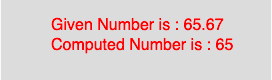GeeksforGeeks App
Open AppBrowser
Continue

## Related Articles

The floor() function in p5.js is used to calculate the floor value of a number. This function maps to the Math.floor() of javascript. It calculates the closest int value that is less than or equal to the value of the parameter.

Syntax

```floor(number)
```

Parameters: The function accepts only one parameter as mentioned above and described below:

• number : This parameter stores the number to compute.
• Below program illustrates the floor() function in p5.js:
Example:

 `function` `setup() {`` ` `    ``//create Canvas of size 270*80  ``    ``createCanvas(270, 80);``}`` ` `function` `draw() {``    ``background(220);``    ``//initialize the parameter  ``    ``let x = 65.67;``    ``//call to floor() function  ``    ``let y = floor(x);``    ``textSize(16);``    ``fill(color(``'red'``));``    ``text(``"Given Number is : "` `+ x, 50, 30);``    ``text(``"Computed Number is : "` `+ y, 50, 50);``}`

Output:Reference: https://p5js.org/reference/#/p5/floor

My Personal Notes arrow_drop_up
Related Tutorials